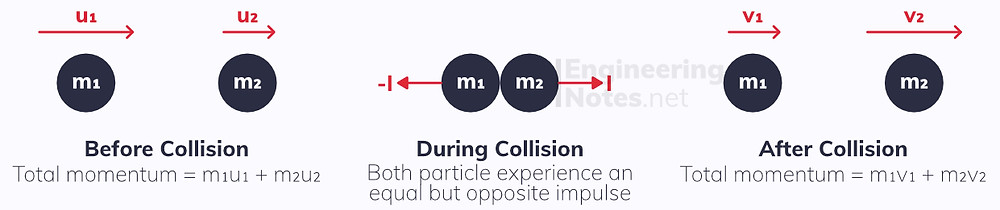#### Notes by Category University Engineering

Electronics*
Mathematics*
Mechanics & Stress Analysis*
Rate these notesNot a fanNot so goodGoodVery goodBrillRate these notes
• A-Level Further Maths

# Momentum, Impulse, Work, Energy & Power

As you know, momentum, p, is defined as the product of an object's mass and velocity:

p = mv

### The Impulse-Momentum Principle

When an object collides with something else, it experiences a change in momentum. This is equal to the impulse experienced by that object in the collision:

I = Δmv

The impulse can also be calculated as the product of the force of the collision, F, and its duration, t:

I = Δmv = Ft
• Therefore, the units of impulse are Newton-seconds, Ns.

Often, the force will have to be calculated using Newton's second law (F=ma), or SUVAT equations need to be used to calculate the duration or change in momentum.

### The Principle of Conservation of Momentum

According to Newton's third law, for every reaction there is an equal but opposite reaction. This means that when two particles collide, both experience the same magnitude of impulse, but in opposite directions. Since impulse equals the change in momentum, the two particles experience equal and opposite changes in momentum, Δp and -Δp. Overall, these two changes in momentum cancel out:

the total momentum before impact equals the total momentum after the impactMathematically, this can be expressed as:

m₁u₁ + m₂u₂ = m₁v₁ + m₂v₂

## Work & Energy

Work done is defined as the the amount of energy transferred from one form to another when a force causes movement. This is known as the work-energy principle.

W = Fx Work done = force x distance moved.
• The force and motion must be in the same direction

Because of this, you will often need to use trigonometry to find the correct components of forces: